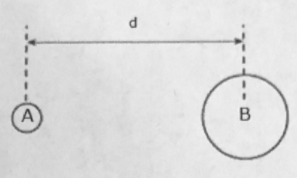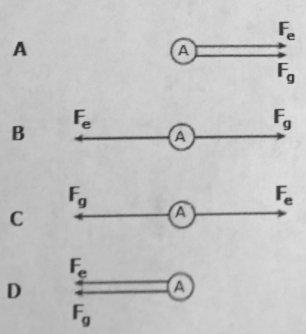# Problem: In the diagram below, two positively charged spheres, A and B, of masses mA and mB, are separated by a distance, dWhich of the following diagrams best represents the direction of the gravitational force, Fg, and the electrostatic force, Fe, acting on sphere A due to the mass and charge of sphere B?

###### FREE Expert Solution

In this problem, we are required to apply the concepts presented in Newton's law of universal gravitation and the coulomb's law.

Coulomb's force is repulsive for like charges and attractive for unlike charges

90% (128 ratings)###### Problem Details

In the diagram below, two positively charged spheres, A and B, of masses mA and mB, are separated by a distance, dWhich of the following diagrams best represents the direction of the gravitational force, Fg, and the electrostatic force, Fe, acting on sphere A due to the mass and charge of sphere B?Frequently Asked Questions

What scientific concept do you need to know in order to solve this problem?

Our tutors have indicated that to solve this problem you will need to apply the Coulomb's Law (Electric Force) concept. You can view video lessons to learn Coulomb's Law (Electric Force). Or if you need more Coulomb's Law (Electric Force) practice, you can also practice Coulomb's Law (Electric Force) practice problems.

What professor is this problem relevant for?

Based on our data, we think this problem is relevant for Professor Chu & Deng's class at UH.